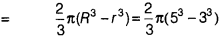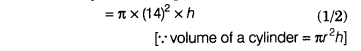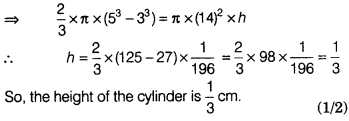# A hemispherical bowl of internal and external diameters 6 cm and 10 cm, respectively

A hemispherical bowl of internal and external diameters 6 cm and 10 cm, respectively is melted and formed into a right circular cylinder of radius 14 cm. Find the height of the cylinder.

Given, outer diameter of the hemispherical bowl
(D)=10 cm
∴ Outer radius of the hemispherical bowl
®=10/2 cm=5 cm
and inner diameter of the hemispherical bowl
(d)=6 cm
∴ Inner radius of the hemispherical bowl
®=6/2= 3 cm
Now, volume of the hemispherical bowl=Volume of a hollow hemisphereand volume cylinder of height h and radius 14 cmAccording to the question,
Volume of the hemispherical bowl=Volume of the cylinder